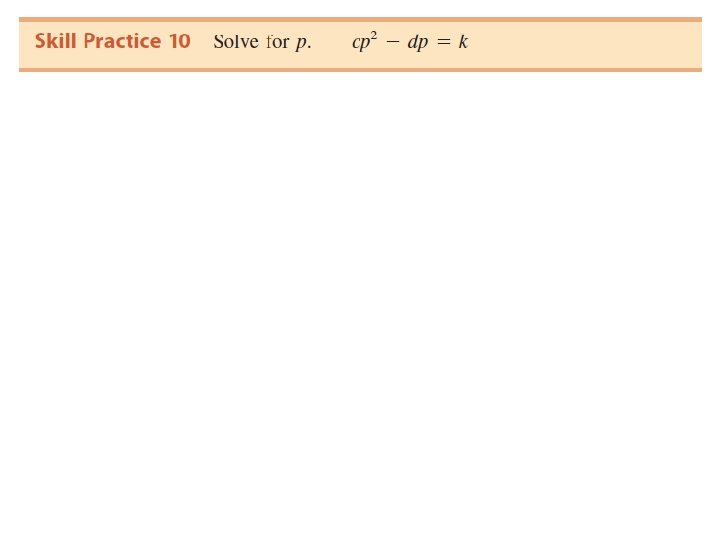• Slides: 39Concepts 1. Solve Quadratic Equations by Using the Zero Product Property 2. Solve Quadratic Equations by Using the Square Root Property 3. Complete the Square 4. Solve Quadratic Equations by Using the Quadratic Formula 5. Use the Discriminant 6. Solve an Equation for a Specified Variable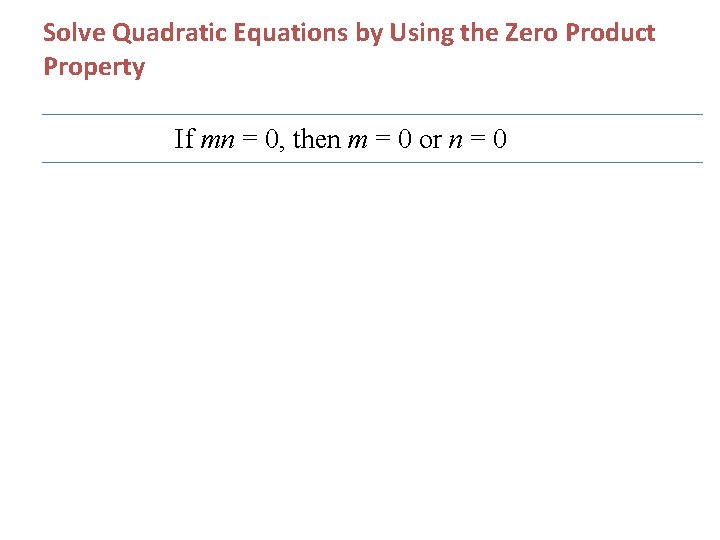Solve Quadratic Equations by Using the Zero Product Property If mn = 0, then m = 0 or n = 0Example 1: SolveExample 2: Solve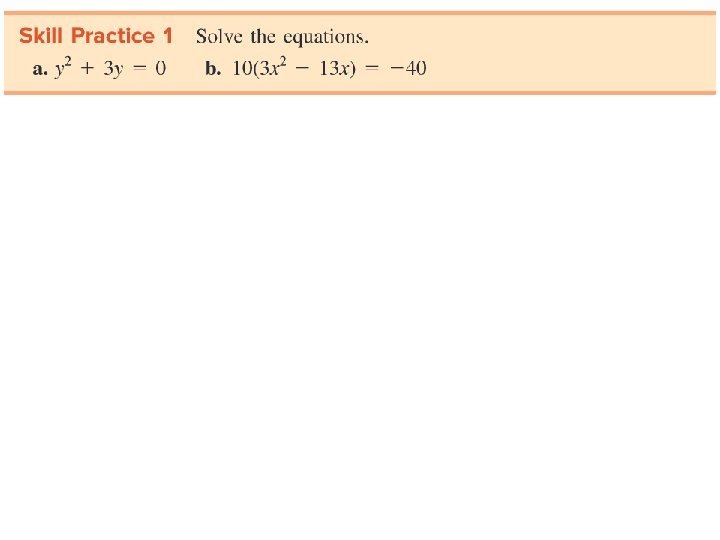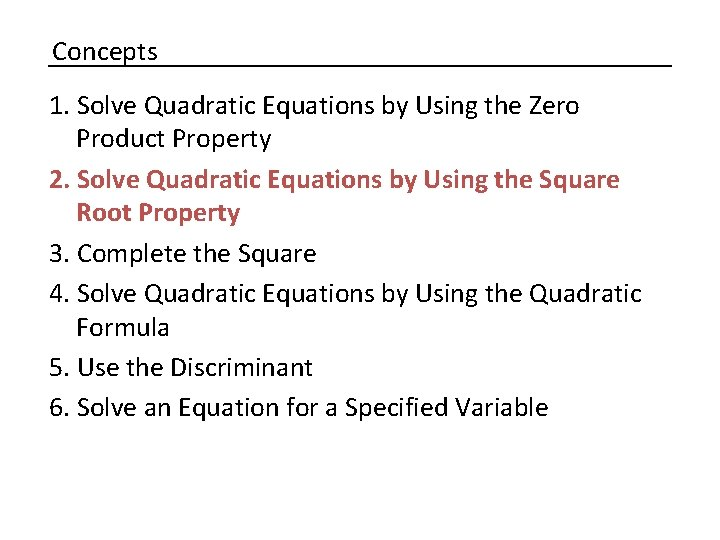Concepts 1. Solve Quadratic Equations by Using the Zero Product Property 2. Solve Quadratic Equations by Using the Square Root Property 3. Complete the Square 4. Solve Quadratic Equations by Using the Quadratic Formula 5. Use the Discriminant 6. Solve an Equation for a Specified VariableSolve Quadratic Equations by Using the Square Root PropertyExample 3: SolveExample 4: Solve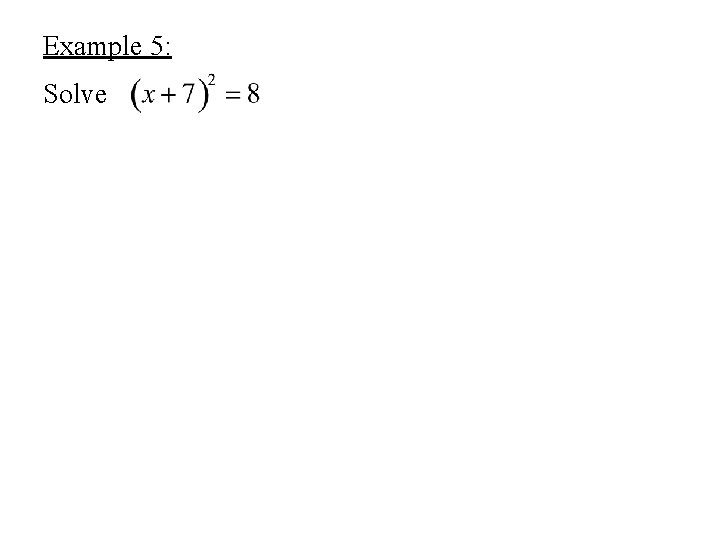Example 5: Solve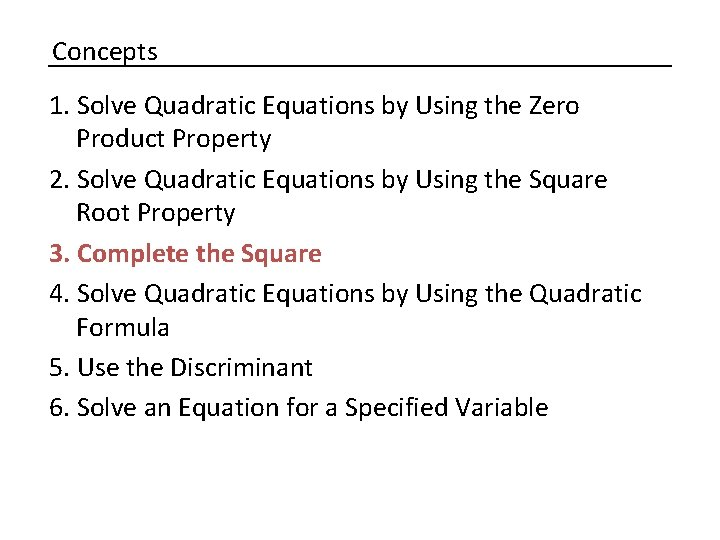Concepts 1. Solve Quadratic Equations by Using the Zero Product Property 2. Solve Quadratic Equations by Using the Square Root Property 3. Complete the Square 4. Solve Quadratic Equations by Using the Quadratic Formula 5. Use the Discriminant 6. Solve an Equation for a Specified VariableComplete the Square Step 1 Divide both sides by a to make the leading coefficient 1. Step 2 Isolate the variable terms on one side of the equation. Step 3 Complete the square. Add the square of one-half the linear term coefficient to both sides. Factor. Step 4 Apply the square root property and solve for x.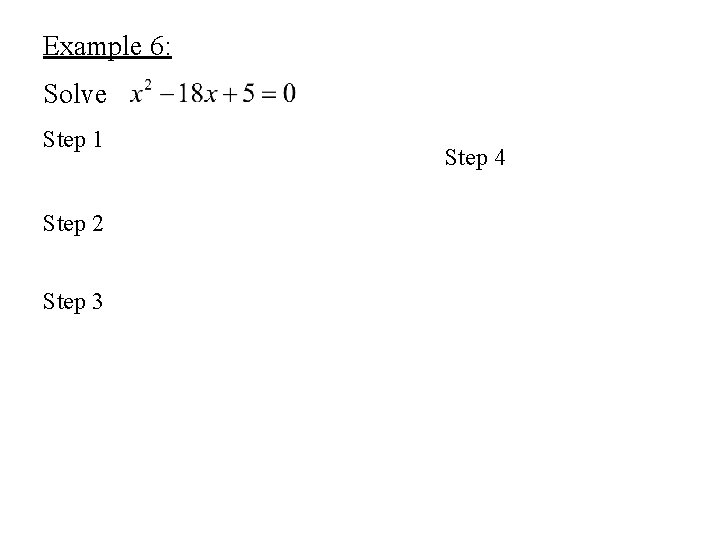Example 6: Solve Step 1 Step 2 Step 3 Step 4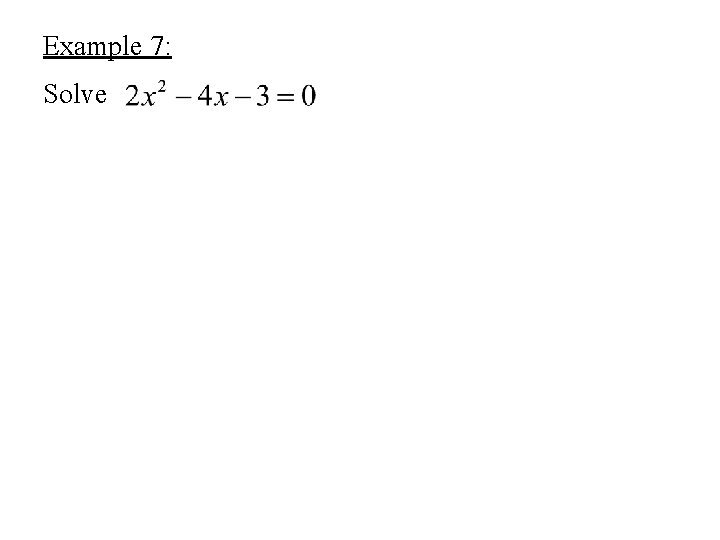Example 7: Solve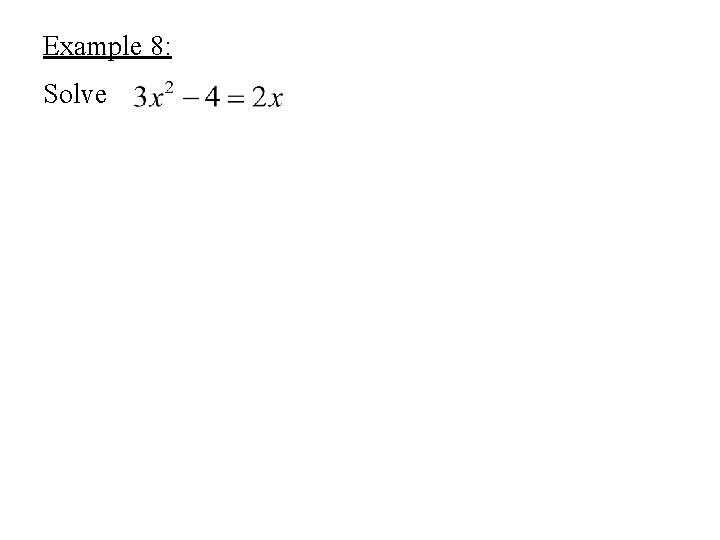Example 8: Solve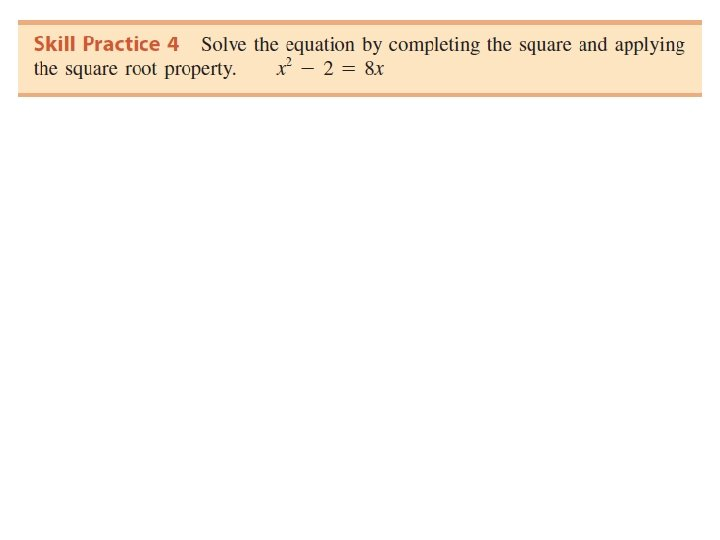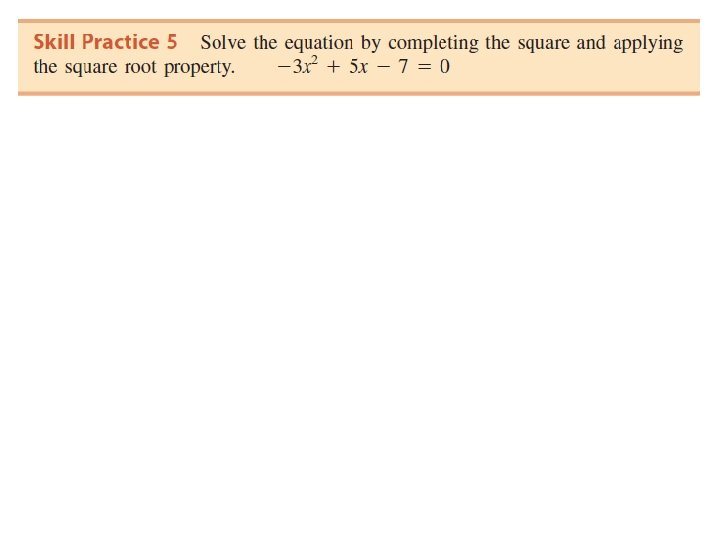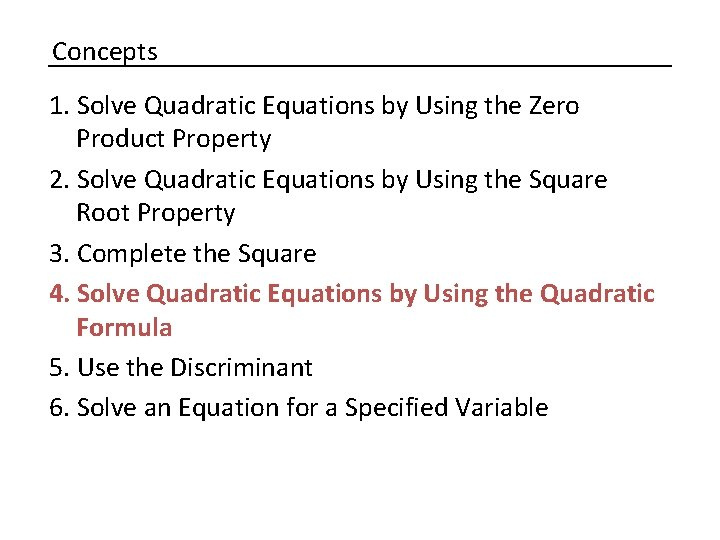Concepts 1. Solve Quadratic Equations by Using the Zero Product Property 2. Solve Quadratic Equations by Using the Square Root Property 3. Complete the Square 4. Solve Quadratic Equations by Using the Quadratic Formula 5. Use the Discriminant 6. Solve an Equation for a Specified Variable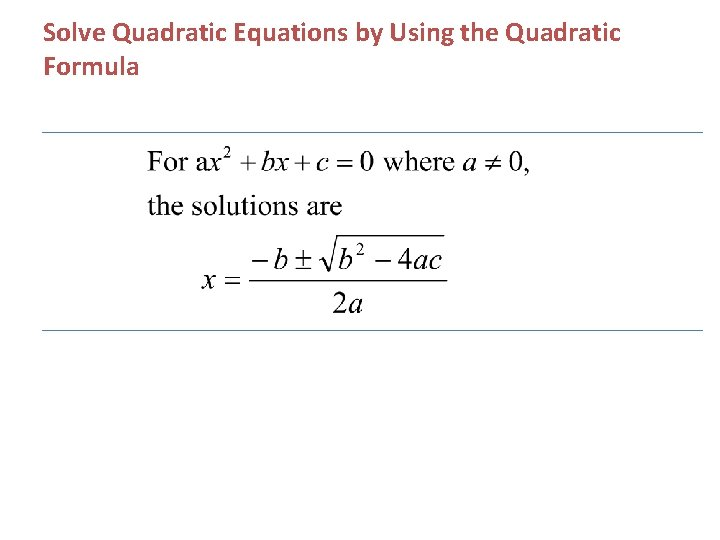Example 9: SolveExample 9 continued: Solve (compare to completing the square)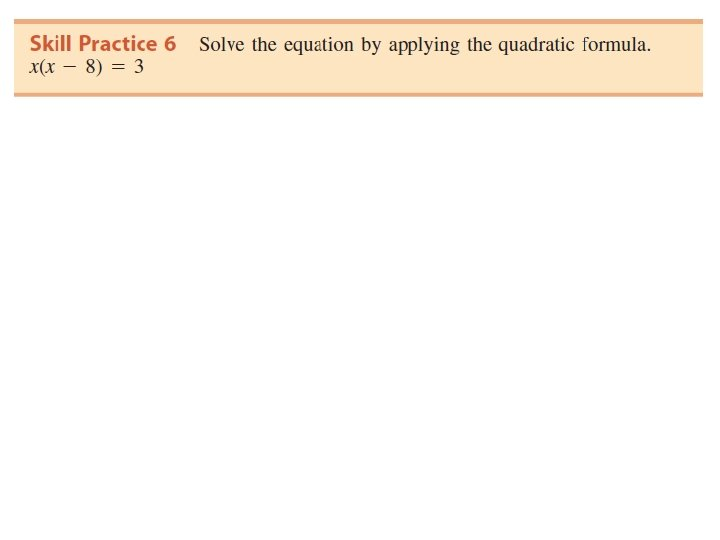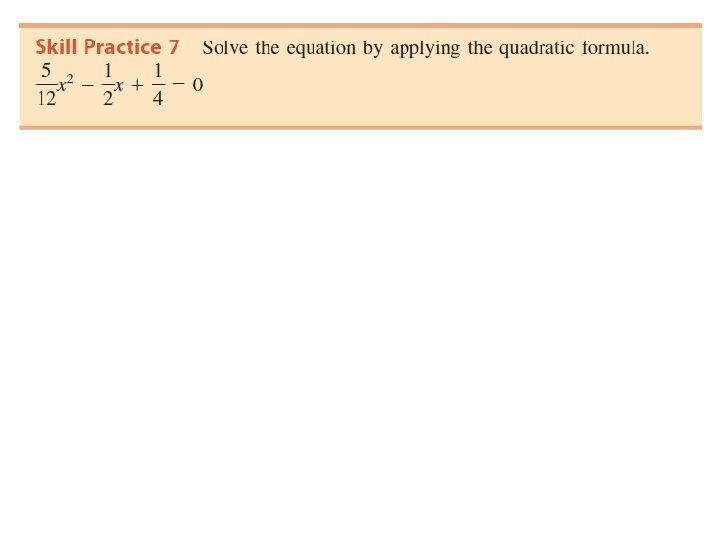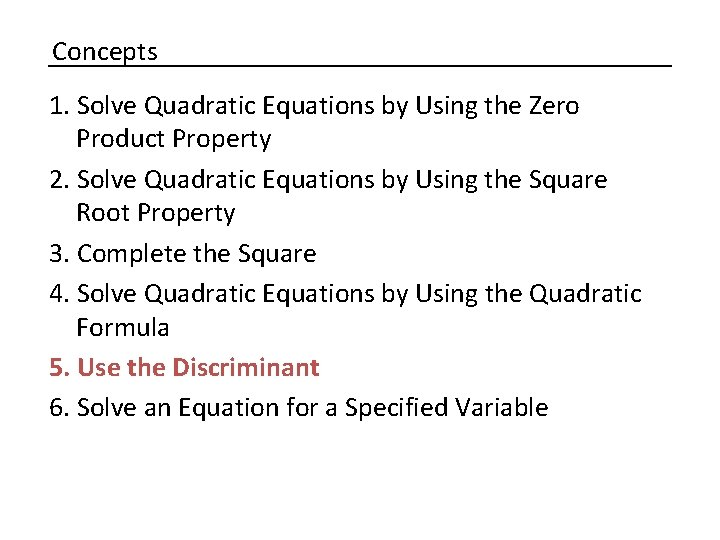Concepts 1. Solve Quadratic Equations by Using the Zero Product Property 2. Solve Quadratic Equations by Using the Square Root Property 3. Complete the Square 4. Solve Quadratic Equations by Using the Quadratic Formula 5. Use the Discriminant 6. Solve an Equation for a Specified Variable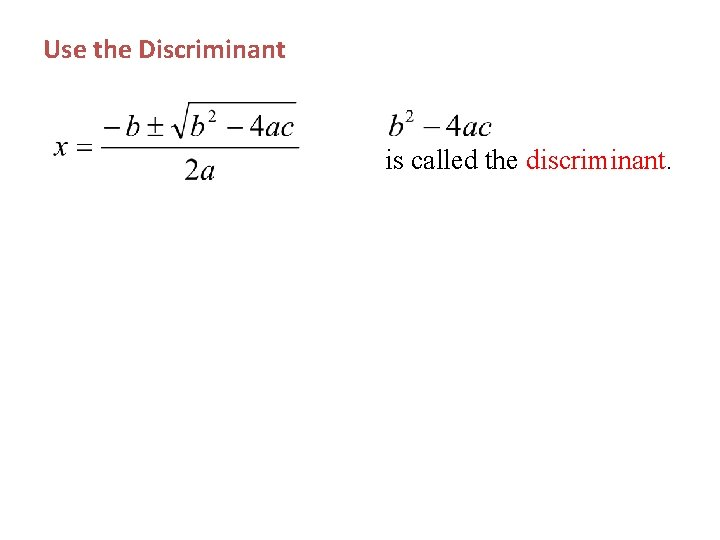Use the Discriminant is called the discriminant.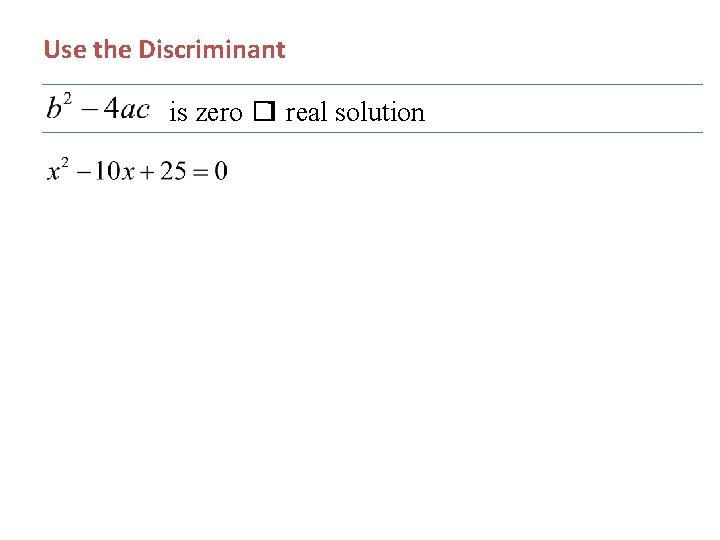Use the Discriminant is zero � 1 real solution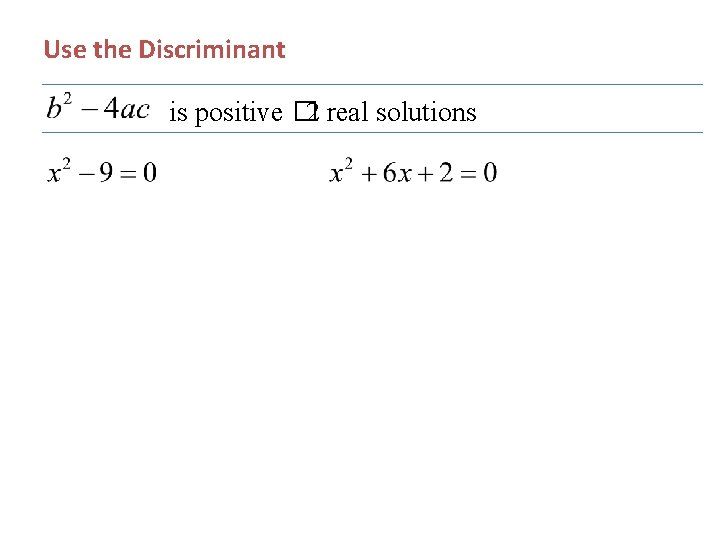Use the Discriminant is positive � 2 real solutions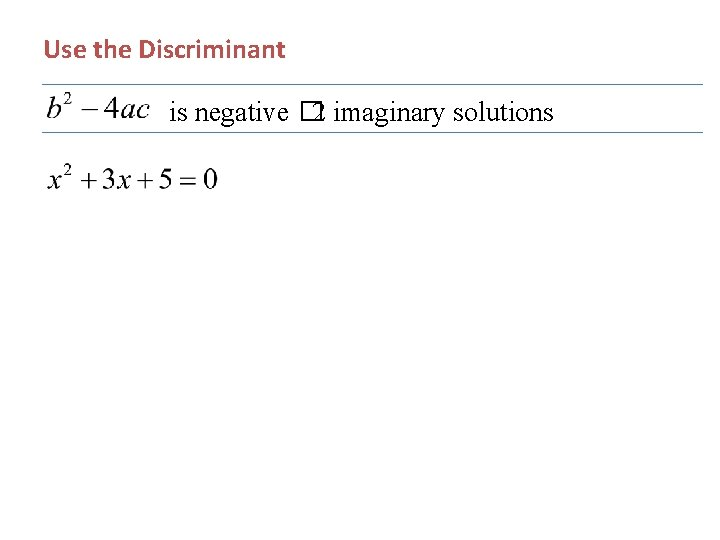Use the Discriminant is negative � 2 imaginary solutions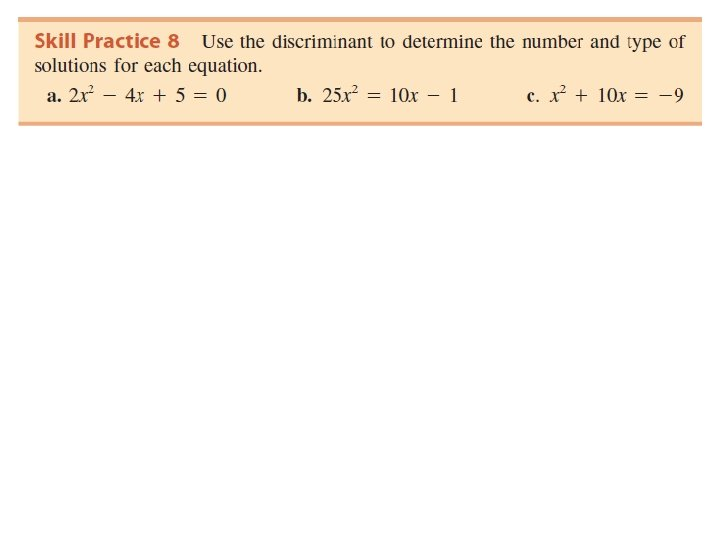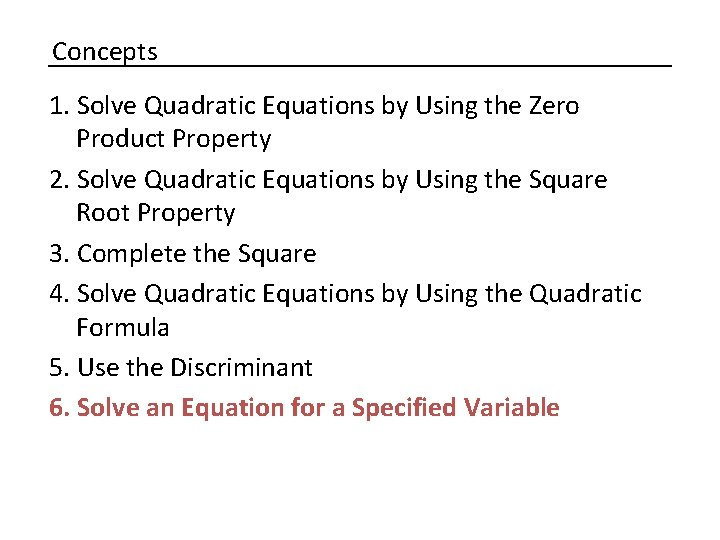Concepts 1. Solve Quadratic Equations by Using the Zero Product Property 2. Solve Quadratic Equations by Using the Square Root Property 3. Complete the Square 4. Solve Quadratic Equations by Using the Quadratic Formula 5. Use the Discriminant 6. Solve an Equation for a Specified Variable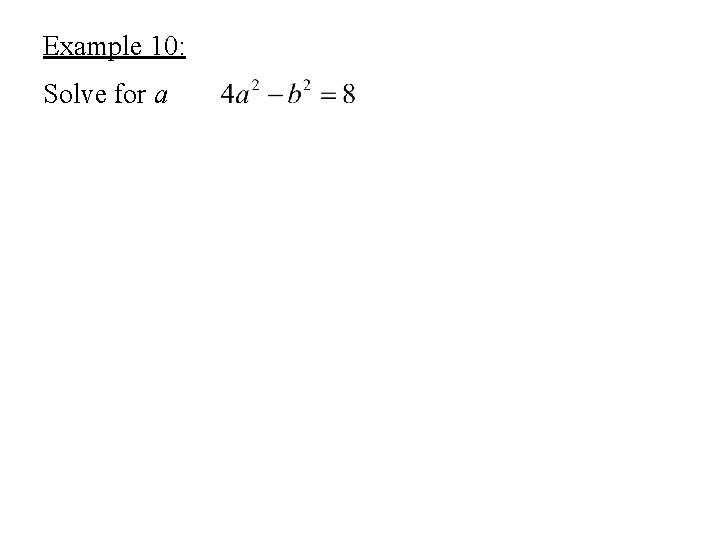Example 10: Solve for aExample 11: Solve for x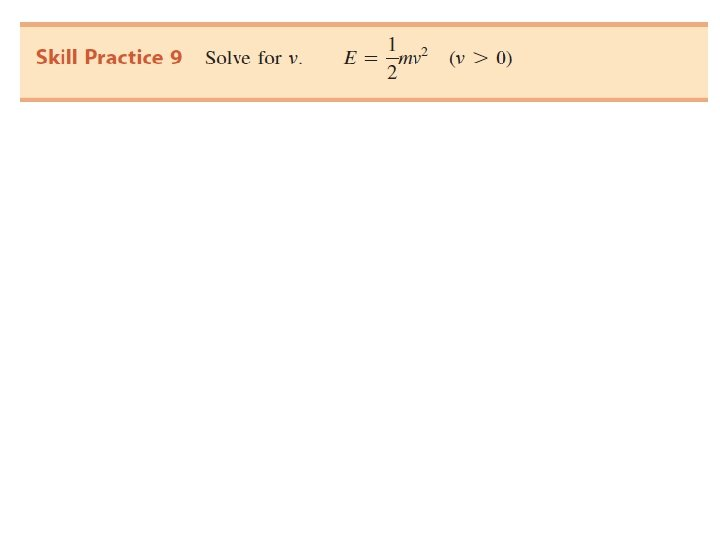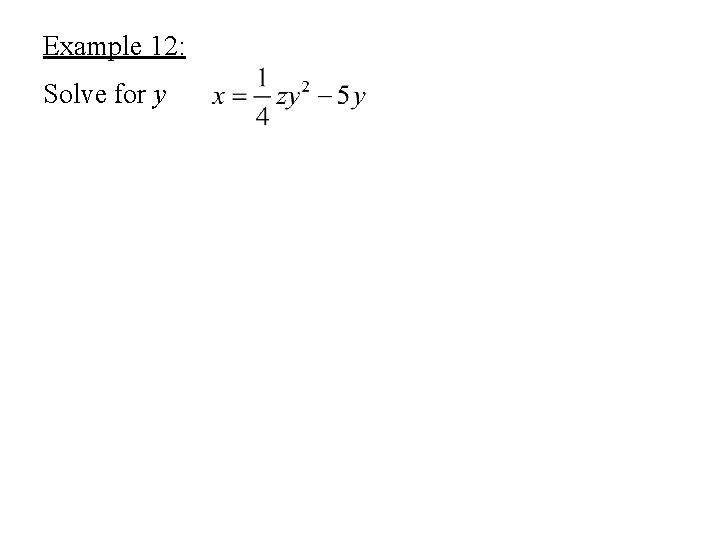Example 12: Solve for yExample 12 continued: